Copyright © Michael Richmond. This work is licensed under a Creative Commons License.

## Vectors and Vector Arithmetic

• Scalar quantities have a magnitude only: examples are mass, temperature, length, time, energy.
• Vector quantities have a magnitude and a direction: examples are velocity ("50 m/s east"), force ("12 newtons up"), displacement ("18 inches to the left").
• Vectors are identified either by boldface type, or by an arrow over the symbol.
• One may also express vectors as a set of components:
```            vector A   =  ( Ax , Ay )
```
• Vector arithmetic is not as simple as scalar arithmetic: the results depend not only on the magnitudes of the inputs, but also on their directions.
• To add or subtract vectors, one may use a graphical method. Place the vectors head-to-tail, and the difference between the tail of the first and the head of the last is the vector sum.
• One may also add or subtract vectors by breaking each down into components, then adding or subtracting the component values:
```            vector A   =  ( 12,  5 )
vector B   =  ( -4, 20 )

x component        y component
--------------------------------------
A + B          12 + (-4)            5 + 20
8                  25

vector (A+B)   =  (  8, 25 )
```
• One can use trigonometry to calculate the components of a vector, or to calculate the magnitude and direction of a vector from its components.

Viewgraphs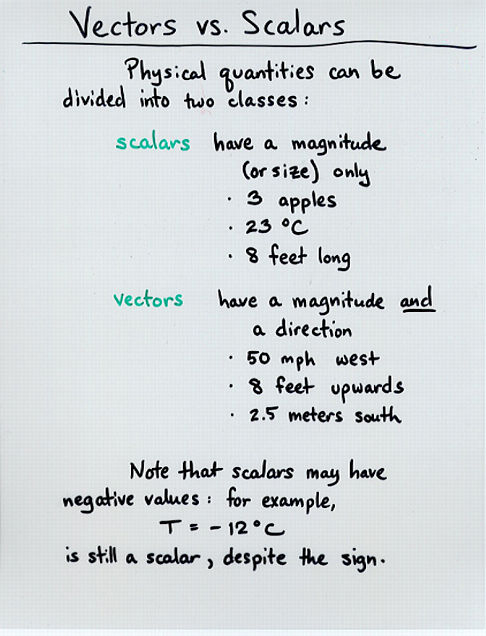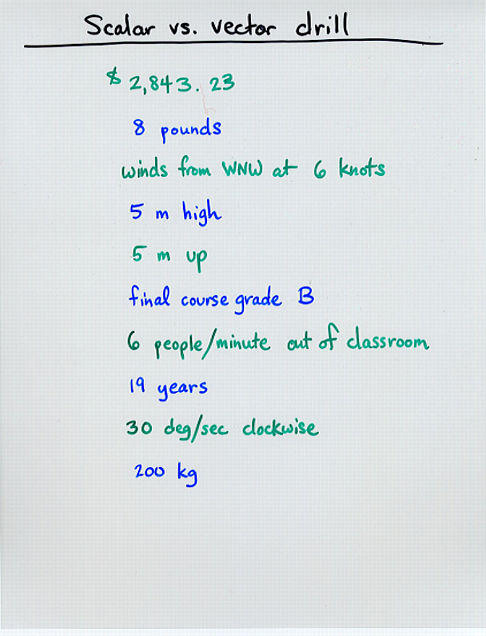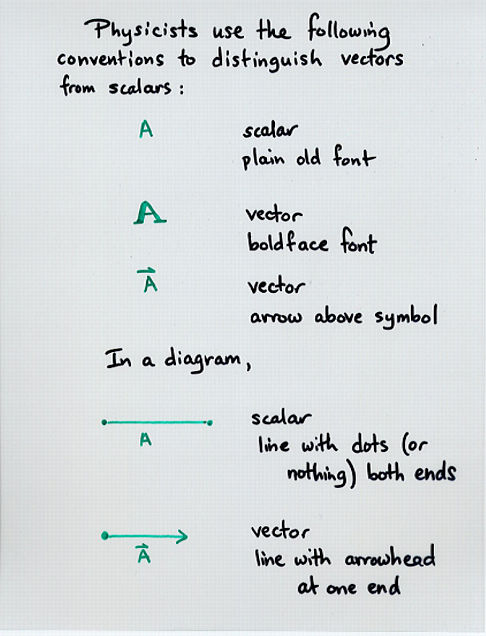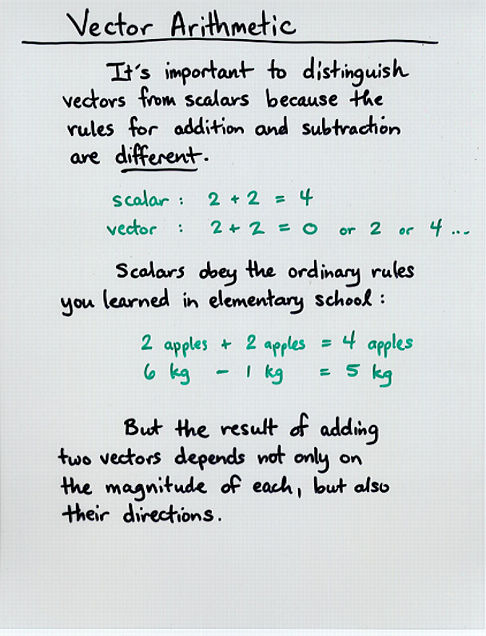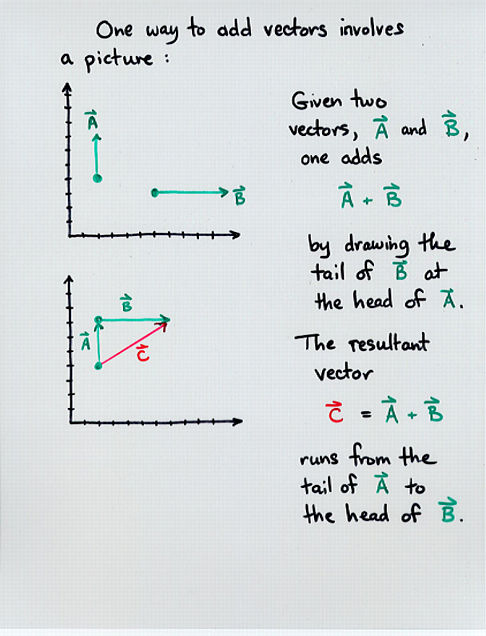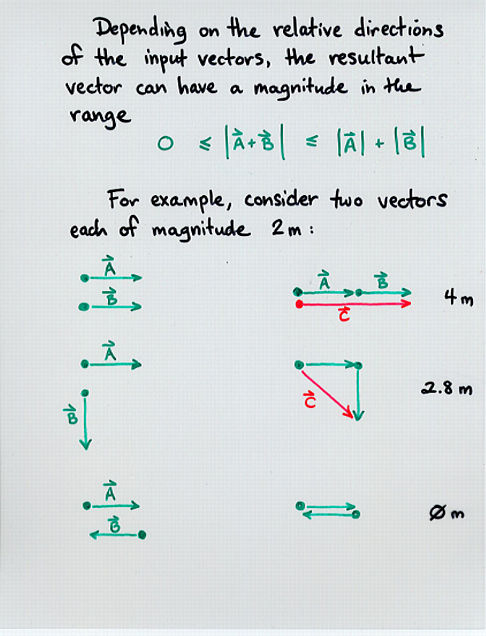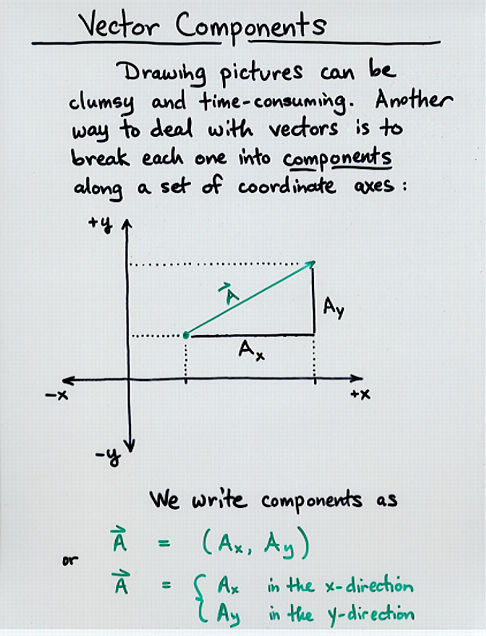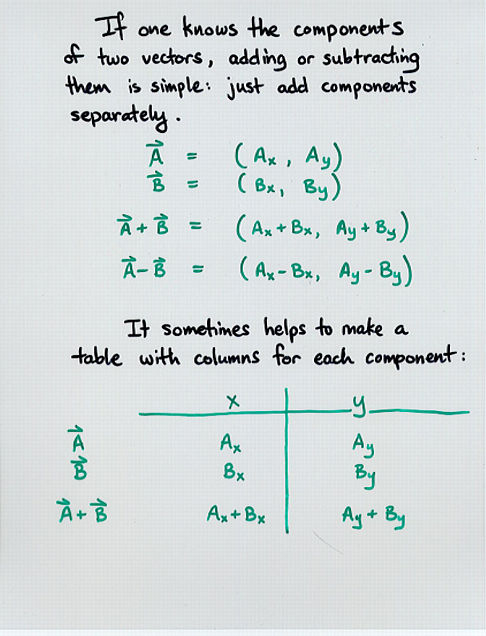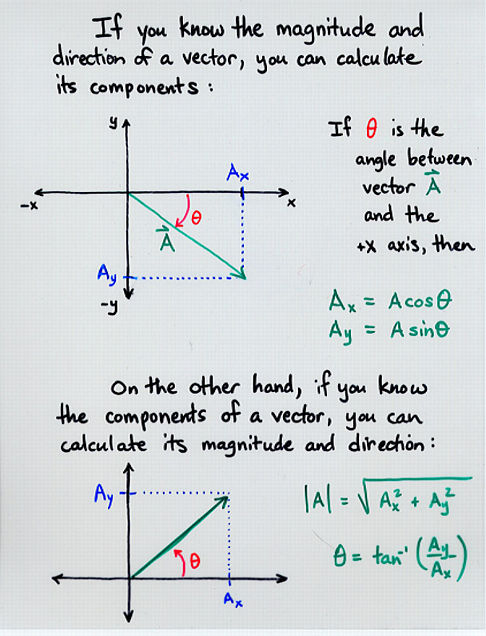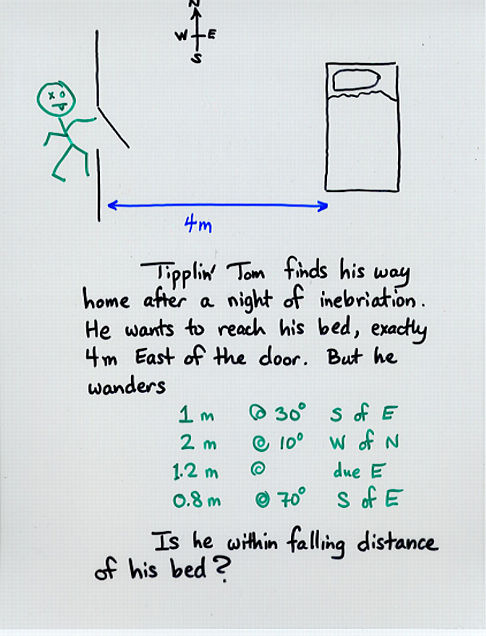Copyright © Michael Richmond. This work is licensed under a Creative Commons License.